# Chapter 5 Graphs with ggplot2

## 5.1 Summary

In this session, we’ll first learn how to read some external data (from .xls, .xlsx, and CSV files) into R with the `readr` and `readxl` packages (both part of the `tidyverse`).

Then, we’ll write reproducible code to build graphs piece-by-piece. In Excel, graphs are made by manually selecting options - which, as we’ve discussed previously, may not be the best option for reproducibility. Also, if we haven’t built a graph with reproducible code, then we might not be able to easily recreate a graph or use that code again to make the same style graph with different data.

Using `ggplot2`, the graphics package within the `tidyverse`, we’ll write reproducible code to manually and thoughtfully build our graphs.

“ggplot2 implements the grammar of graphics, a coherent system for describing and building graphs. With ggplot2, you can do more faster by learning one system and applying it in many places.” - R4DS

So yeah…that `gg` is from “grammar of graphics.”

We’ll use the `ggplot2` package, but the function we use to initialize a graph will be `ggplot`, which works best for data in tidy format (i.e., a column for every variable, and a row for every observation). Graphics with `ggplot` are built step-by-step, adding new elements as layers with a plus sign (`+`) between layers (note: this is different from the pipe operator, `%>%`. Adding layers in this fashion allows for extensive flexibility and customization of plots.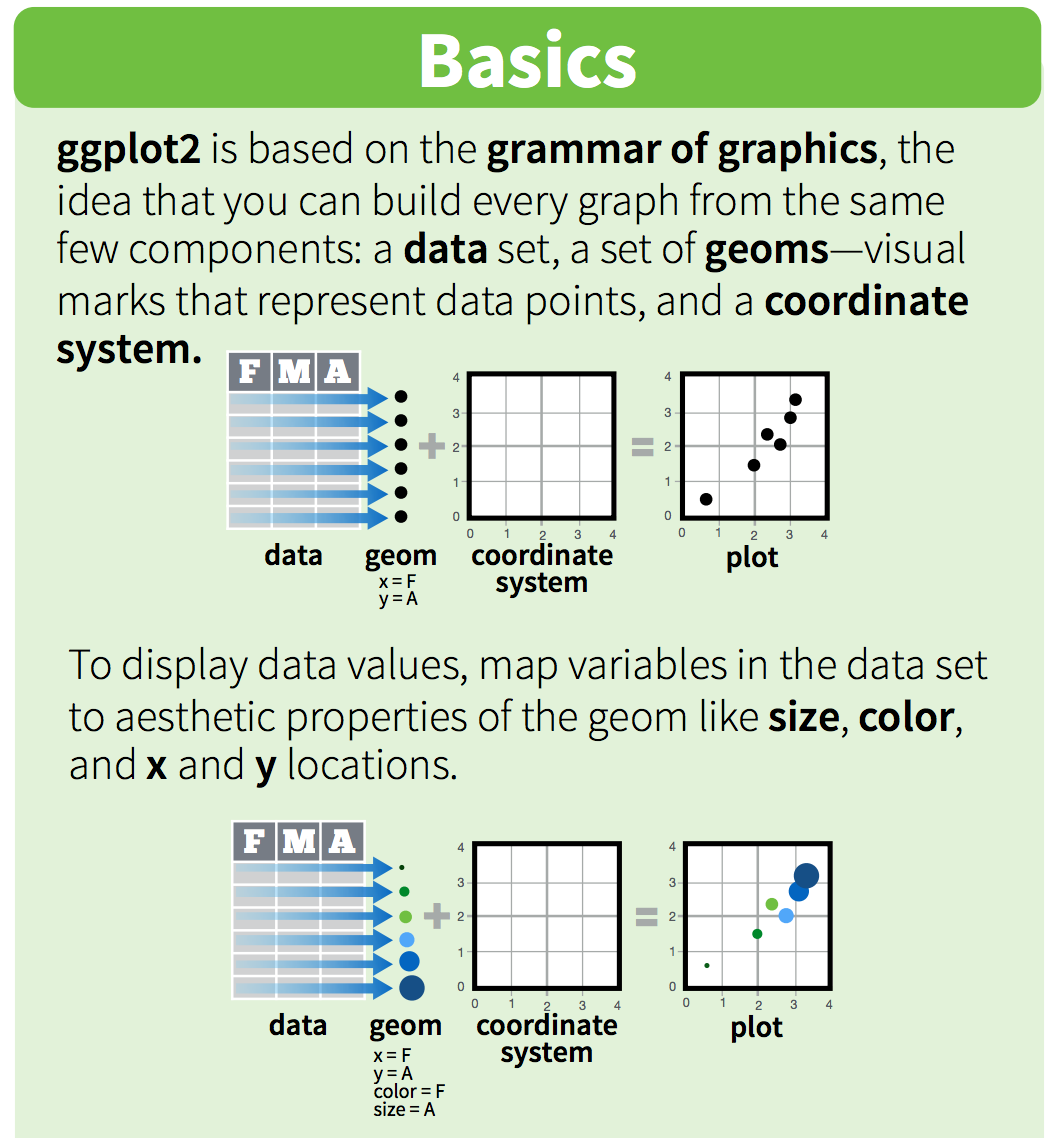### 5.1.1 Objectives

• Read in external data (Excel files, CSVs) with `readr` and `readxl`
• Initial data exploration
• Build several common types of graphs (scatterplot, column, line) in ggplot2
• Customize gg-graph aesthetics (color, style, themes, etc.)
• Update axis labels and titles
• Combine compatible graph types (geoms)
• Build multiseries graphs
• Split up data into faceted graphs
• Export figures with `ggsave()`

## 5.2 Getting started - In existing .Rmd, attach packages

In your existing `plots-ggplot.Rmd` from Session 2, remove everything below the first code chunk.

The `ggplot2` package is part of the `tidyverse`, so we don’t need to attach it separately. Attach the `tidyverse`, `readxl` and `here` packages in the top-most code chunk of your .Rmd.

``````library(tidyverse)
library(readxl)
library(here)``````

## 5.3 Read in .xlsx and .csv files

In this session, we’ll use data for parks visitation from two files:

• A comma-separated-value (CSV) file containing visitation data for all National Parks in California (ca_np.csv)
• A single Excel worksheet containing only visitation for Channel Islands National Park (ci_np.xlsx)

### 5.3.1`read_csv()` to read in comma-separated-value (.csv) files

There are many types of files containing data that you might want to work with in R. A common one is a comma separated value (CSV) file, which contains values with each column entry separated by a comma delimiter. CSVs can be opened, viewed, and worked with in Excel just like an .xls or .xlsx file - but let’s learn how to get data directly from a CSV into R where we can work with it more reproducibly.

To read in the ca_np.csv file, we need to:

• insert a new code chunk
• use `read_csv()` to read in the file
• use `here()` within `read_csv()` to tell it where to look
• assign the stored data an object name (we’ll store ours as ca_np)
``ca_np <- read_csv(here("data", "ca_np.csv"))``

Look in your Environment to see that ca_np now shows up. Click on the object in the Environment, and R will automatically run the `View()` function for you to pull up your data in a separate viewing tab. Now we can look at it in the spreadsheet format we’re used to.

We can explore our data frame a bit more to see what it contains. For example:

• `names()`: to see the variable (column) names
• `head()`: to see the first x rows (6 is the default)
• `summary()`: see a quick summary of each variable

(Remember that `names()` is the name of the function but `names(ca_np)` is how we use it on the data.)

Cool! Next, let’s read in ci_np.xlsx an Excel file) using `read_excel()`.

### 5.3.2`readxl` to read in Excel files

We also have an Excel file (ci_np.xlsx) that contains observations only for Channel Islands National Park visitation. Both `readr` and `readxl` are part of the `tidyverse`, which means we should expect their functions to have similar syntax and structure.

Note: If `readxl` is part of the `tidyverse`, then why did I have to attach it separately? Great question! Too keep the `tidyverse` manageable, there are “core” packages (`readr`, `dplyr`, `tidyr`, `ggplot2`, `forcats`, `purrr`, `tibble`, `stringr`) that you would expect to use frequently, and those are automatically attached when you use `library(tidyverse)`. But there are also more specialized `tidyverse` packages (e.g. `readxl`, `reprex`, `lubridate`, `rvest`) that are built with similar design philosophy, but are not automatically attached with `library(tidyverse)`. Those specialized packages are installed along with the `tidyverse`, but need to be attached individually (e.g. with `library(readxl)`).

Use `read_excel()` to get the ci_np.xlsx data into R:

``ci_np <- read_excel(here("data", "ci_np.xlsx"))``

Note: If you want to explicitly read in an .xlsx or .xls file, you can use `read_xlsx()` or `read_xls()` instead. `read_excel()` will make its best guess about which type of Excel file you’re reading in, so is the generic form.

Explore the ci_np data frame as above using functions like `View()`, `names()`, `head()`, and `summary()`.

Now that we have read in the National Parks visitation data, let’s use `ggplot2` to visualize it.

## 5.4 Our first ggplot graph: Visitors to Channel Islands NP

To create a bare-bones ggplot graph, we need to tell R three basic things:

1. We’re using `ggplot2::ggplot()`
2. Data we’re using & variables we’re plotting (i.e., what is x and/or y?)
3. What type of graph we’re making (the type of geom)

Generally, that structure will look like this:

``````ggplot(data = df_name, aes(x = x_var_name, y = y_var_name)) +
geom_type()``````

Breaking that down:

• First, tell R you’re using `ggplot()`
• Then, tell it the object name where variables exist (`data = df_name`)
• Next, tell it the aesthetics `aes()` to specify which variables you want to plot
• Then add a layer for the type of geom (graph type) with `geom_*()` - for example, `geom_point()` is a scatterplot, `geom_line()` is a line graph, `geom_col()` is a column graph, etc.

Let’s do that to create a line graph of visitors to Channel Islands National Park:

``````ggplot(data = ci_np, aes(x = year, y = visitors)) +
geom_line()``````We’re going to be doing a lot of plot variations with those same variables. Let’s store the first line as object `gg_base` so that we don’t need to retype it each time:

``gg_base <- ggplot(data = ci_np, aes(x = year, y = visitors))``

Or, we could change that to a scatterplot just by updating the `geom_*`:

``````gg_base +
geom_point()``````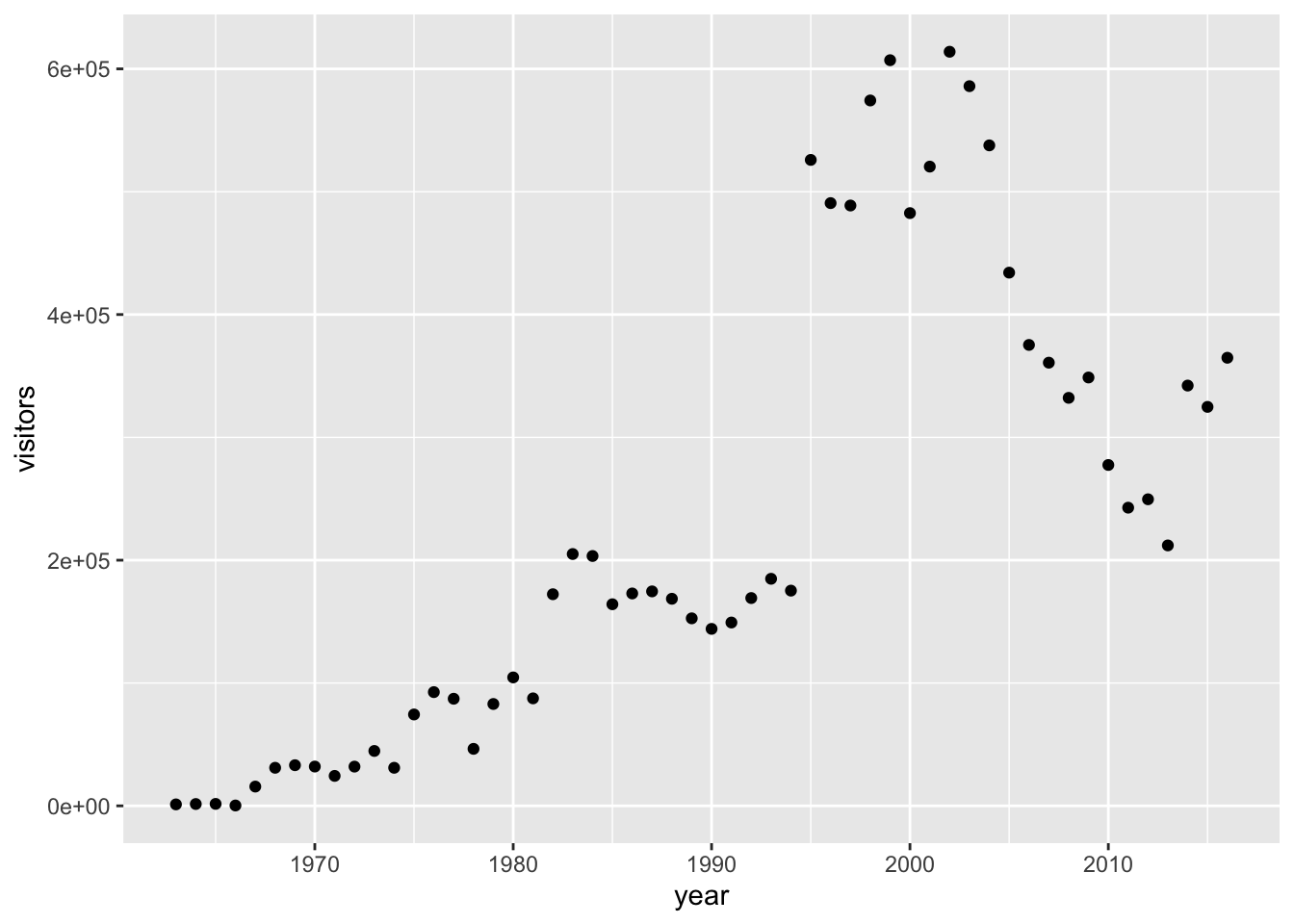We could even do that for a column graph:

``````gg_base +
geom_col()``````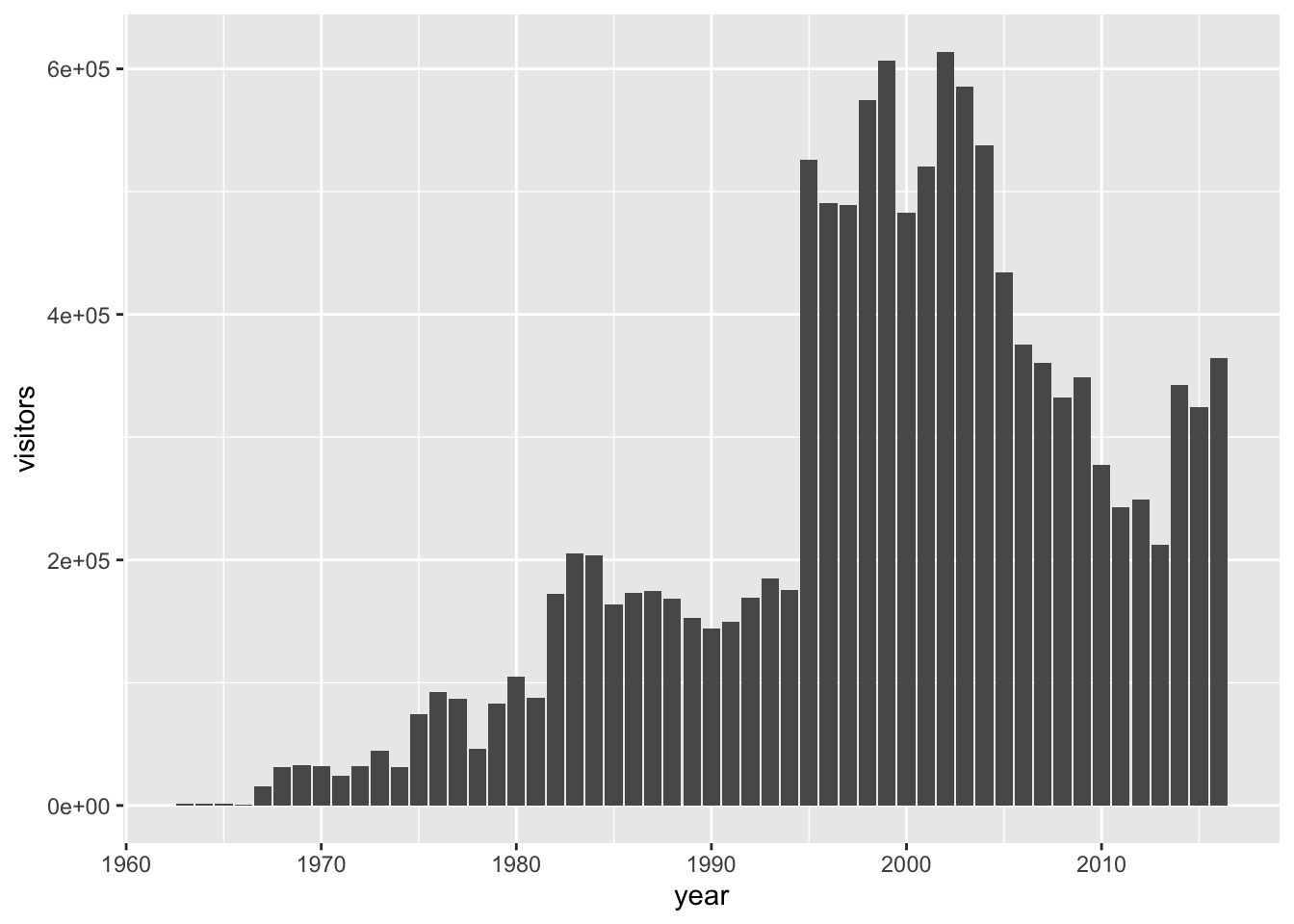Or an area plot…

``````gg_base +
geom_area()``````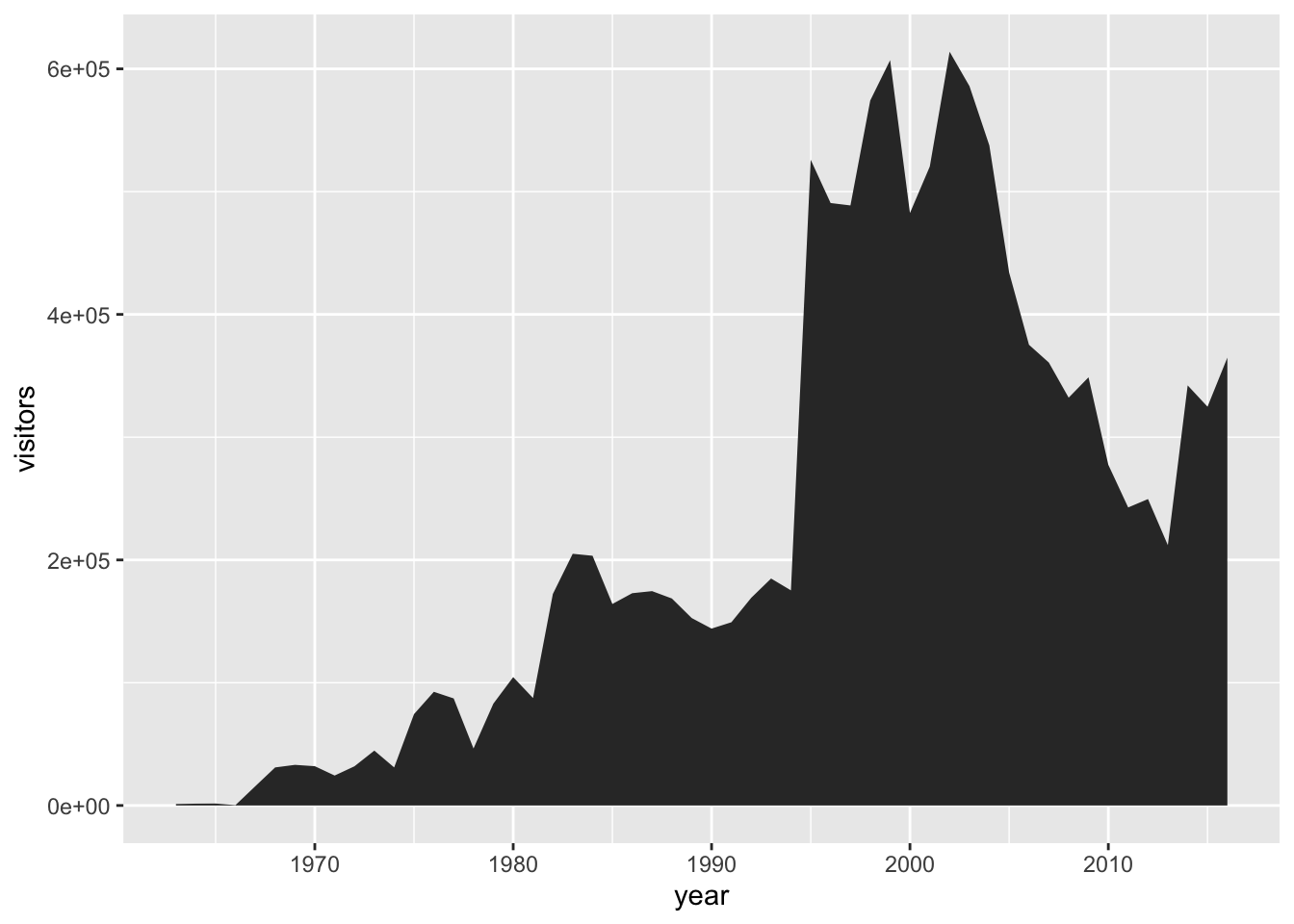We can see that updating to different `geom_*` types is quick, so long as the types of graphs we’re switching between are compatible.

The data are there, now let’s do some data viz customization.

## 5.5 Intro to customizing `ggplot` graphs

First, we’ll customize some aesthetics (e.g. colors, styles, axis labels, etc.) of our graphs based on non-variable values.

We can change the aesthetics of elements in a ggplot graph by adding arguments within the layer where that element is created.

Some common arguments we’ll use first are:

• `color =` or `colour =`: update point or line colors
• `fill =`: update fill color for objects with areas
• `linetype =`: update the line type (dashed, long dash, etc.)
• `pch =`: update the point style
• `size =`: update the element size (e.g. of points or line thickness)
• `alpha =`: update element opacity (1 = opaque, 0 = transparent)

Building on our first line graph, let’s update the line color to “purple” and make the line type “dashed”:

``````gg_base +
geom_line(
color = "purple",
linetype = "dashed"
)``````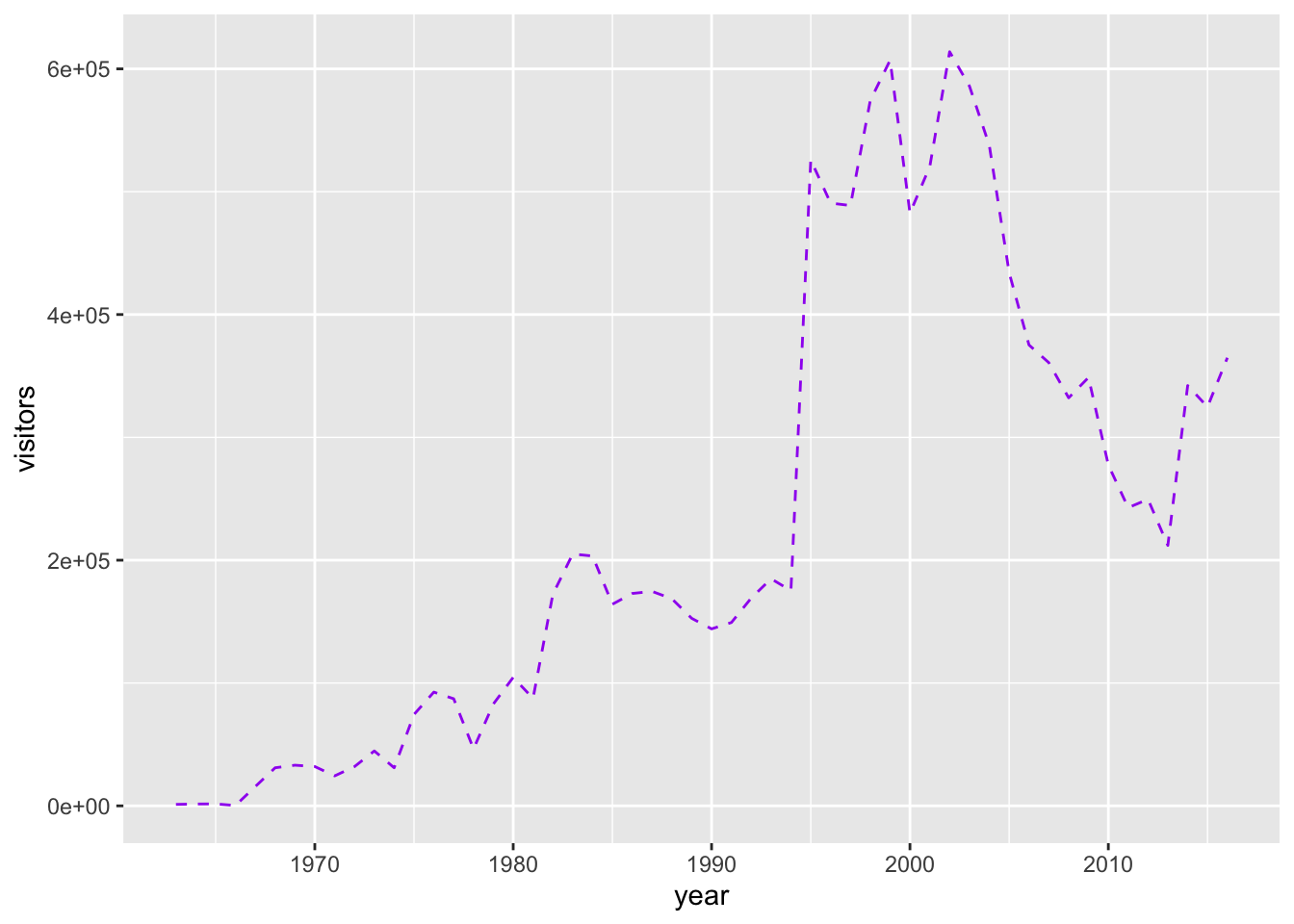How do we know which color names ggplot will recognize? If you google “R colors ggplot2” you’ll find a lot of good resources. Here’s one: SAPE ggplot2 colors quick reference guide

Now let’s update the point, style and size of points on our previous scatterplot graph using `color =`, `size =`, and `pch =` (see `?pch` for the different point styles, which can be further customized).

``````gg_base +
geom_point(color = "purple",
pch = 17,
size = 4,
alpha = 0.5)``````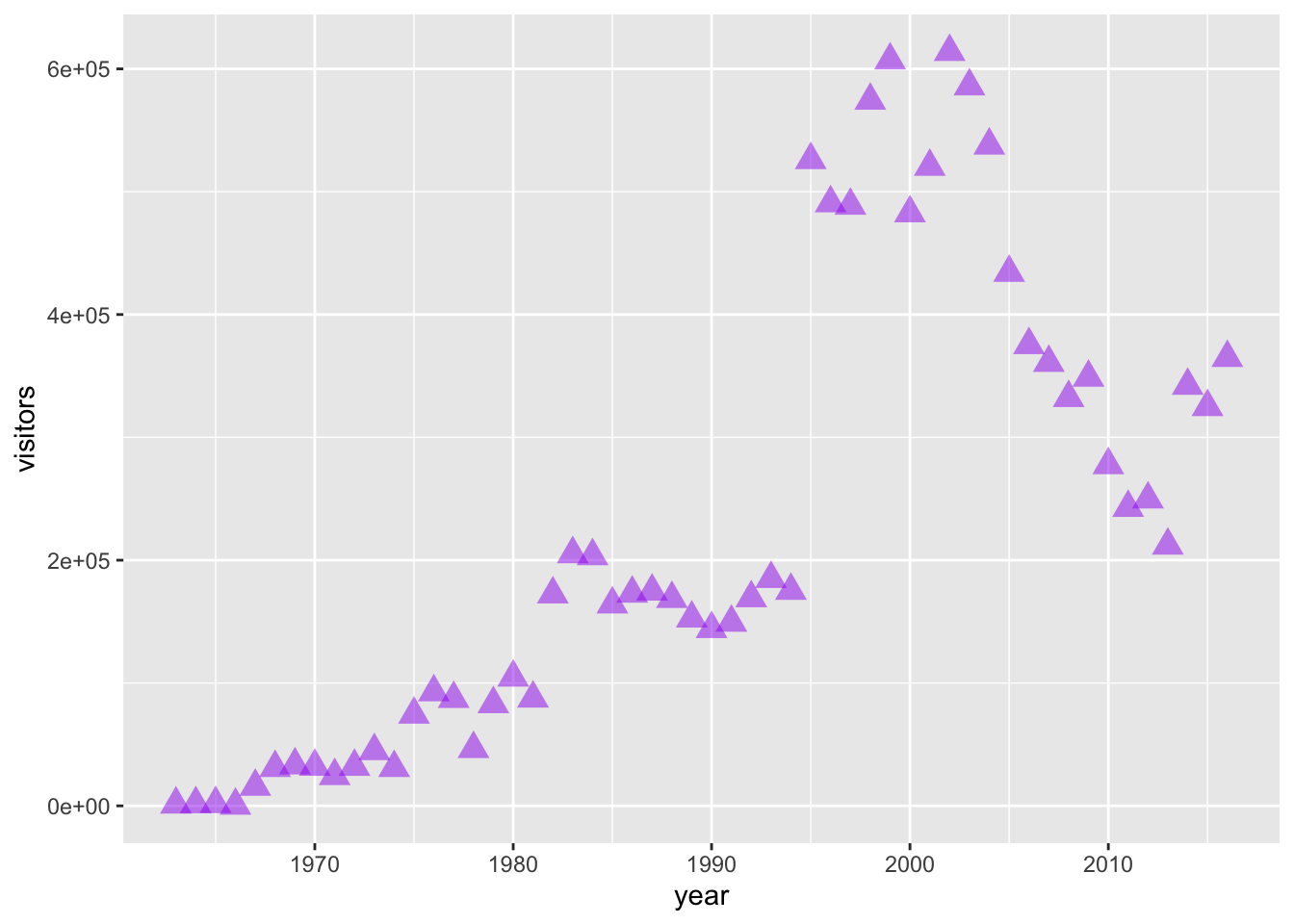### 5.5.1 Activity: customize your own ggplot graph

Update one of the example graphs you created above to customize at least an element color and size!

## 5.6 Mapping variables onto aesthetics

In the examples above, we have customized aesthetics based on constants that we input as arguments (e.g., the color / style / size isn’t changing based on a variable characteristic or value). Sometimes, however, we do want the aesthetics of a graph to depend on a variable. To do that, we’ll map variables onto graph aesthetics, meaning we’ll change how an element on the graph looks based on a variable characteristic (usually, character or value).

When we want to customize a graph element based on a variable’s characteristic or value, add the argument within `aes()` in the appropriate `geom_*()` layer

In short, if updating aesthetics based on a variable, make sure to put that argument inside of `aes()`.

Example: Create a ggplot scatterplot graph where the size and color of the points change based on the number of visitors, and make all points the same level of opacity (`alpha = 0.5`). Notice the `aes()` around the `size =` and `color =` arguments.

Also: this is overmapped and unnecessary. Avoid excessive / overcomplicated aesthetic mapping in data visualization.

``````gg_base +
geom_point(
aes(size = visitors,
color = visitors),
alpha = 0.5
)``````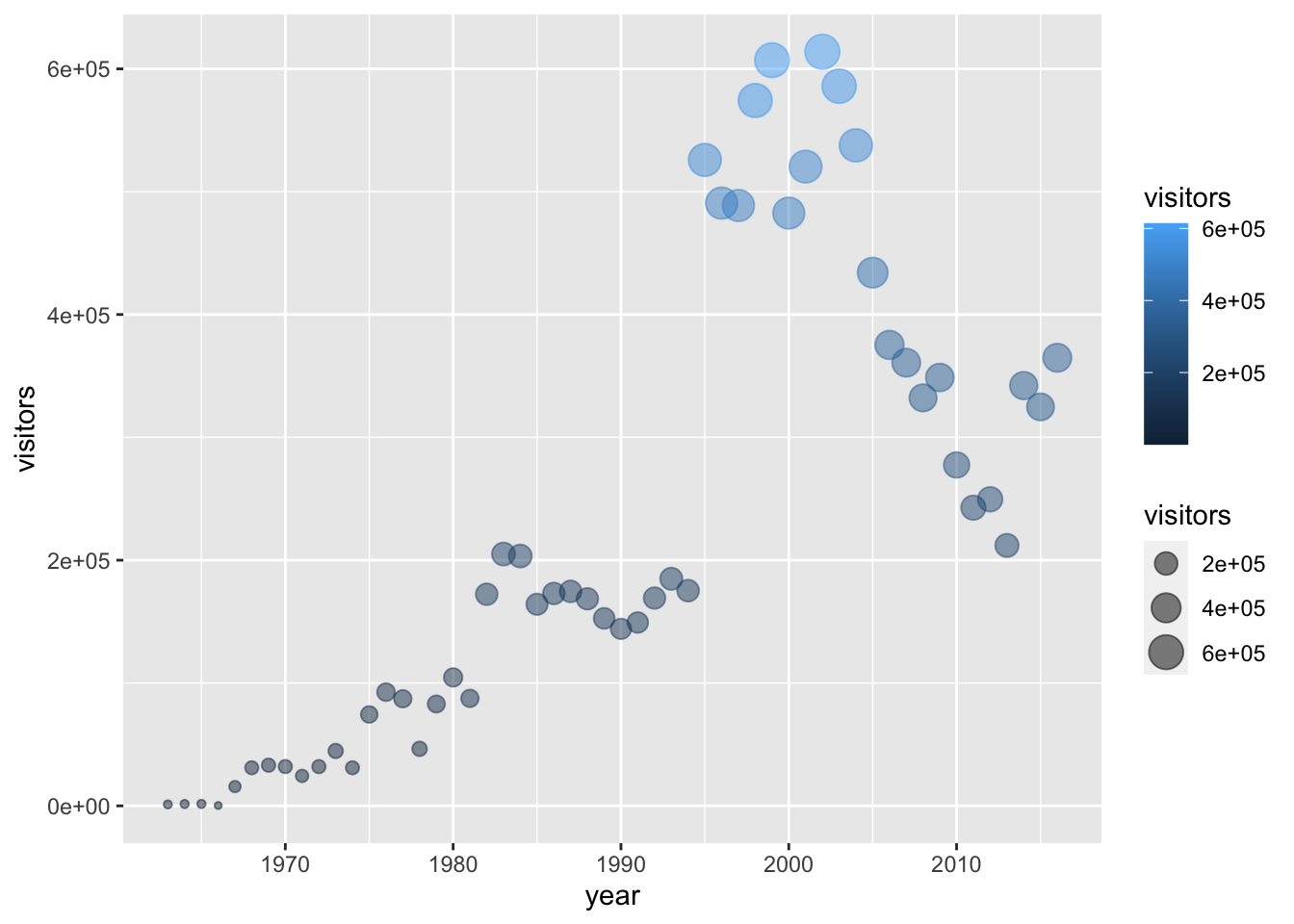In the example above, notice that the two arguments that do depend on variables are within `aes()`, but since `alpha = 0.5` doesn’t depend on a variable then it is outside the `aes()` but still within the `geom_point()` layer.

### 5.6.1 Activity: map variables onto graph aesthetics

Create a column plot of Channel Islands National Park visitation over time, where the fill color (argument: `fill =`) changes based on the number of visitors.

``````gg_base +
geom_col(aes(fill = visitors))``````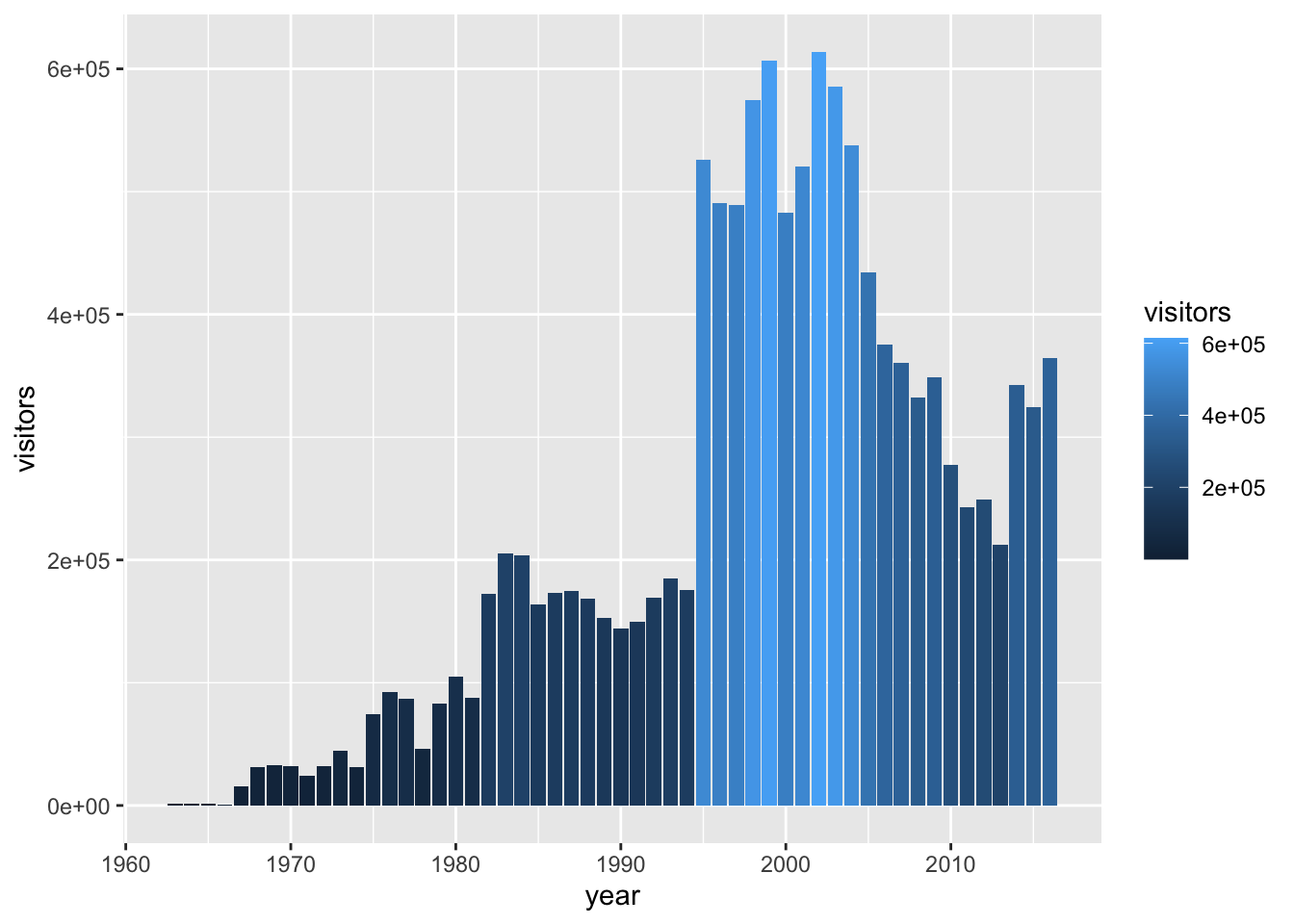Sync your project with your GitHub repo.

## 5.7 ggplot2 complete themes

While every element of a ggplot graph is manually customizable, there are also built-in themes (`theme_*()`) that you can add to your ggplot code to make some major headway before making smaller tweaks manually.

Here are a few to try today (but also notice all the options that appear as we start typing `theme_` into our ggplot graph code!):

• `theme_light()`
• `theme_minimal()`
• `theme_bw()`

Here, let’s update our previous graph with `theme_minimal()`:

``````gg_base +
geom_point(
aes(size = visitors,
color = visitors),
alpha = 0.5
) +
theme_minimal()``````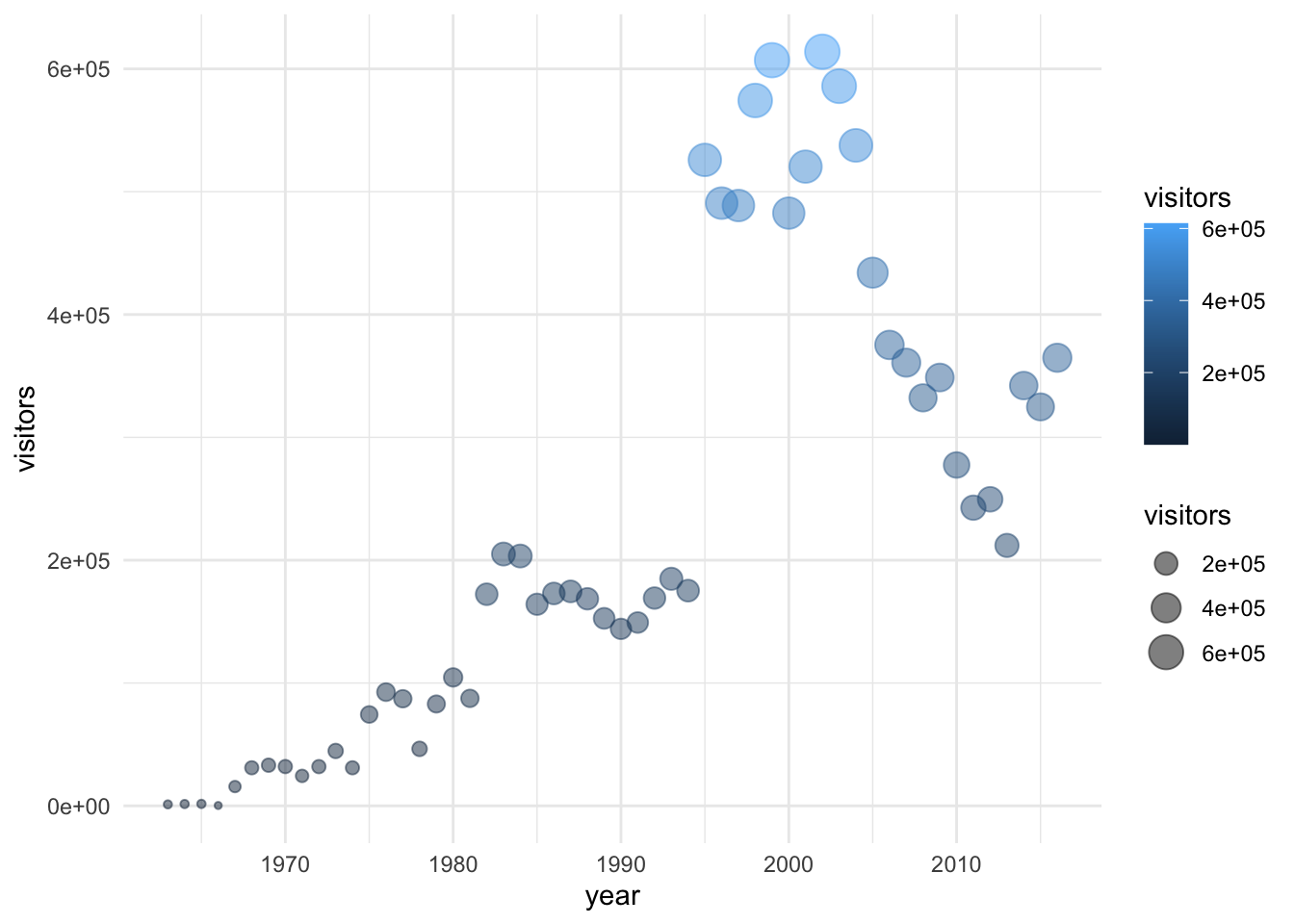## 5.8 Updating axis labels and titles

Use `labs()` to update axis labels, and add a title and/or subtitle to your ggplot graph.

``````gg_base +
geom_line(linetype = "dotted") +
theme_bw() +
labs(
x = "Year",
y = "Annual park visitors",
title = "Channel Islands NP Visitation",
subtitle = "(1963 - 2016)"
)``````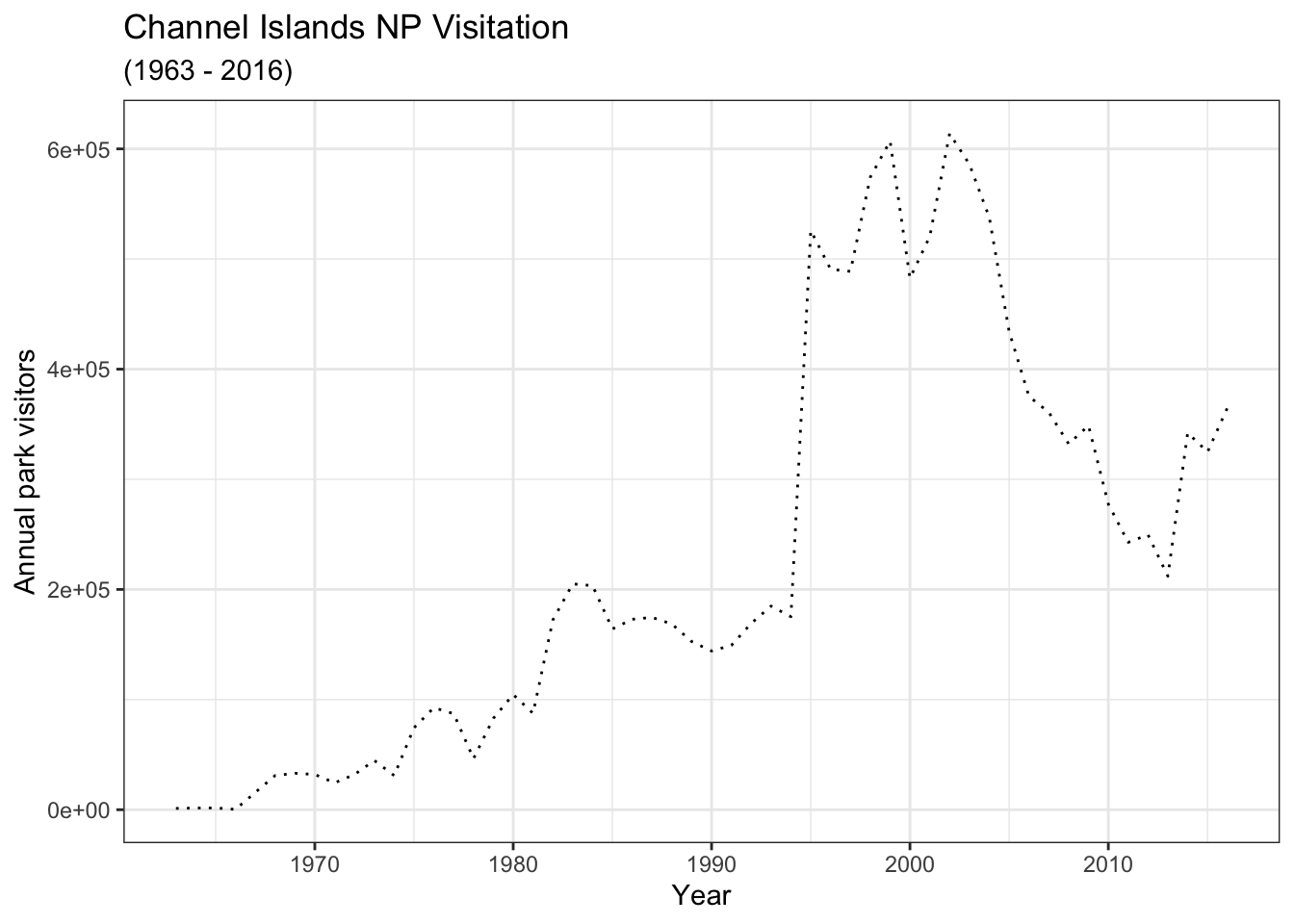Note: If you want to update the formatting of axis values (for example, to convert to comma format instead of scientific format above), you can use the `scales` package options (see more from the R Cookbook).

## 5.9 Combining compatible geoms

As long as the geoms are compatible, we can layer them on top of one another to further customize a graph.

For example, adding points to a line graph:

``````gg_base +
geom_line(color = "purple") +
geom_point(color = "orange",
aes(size = year),
alpha = 0.5)``````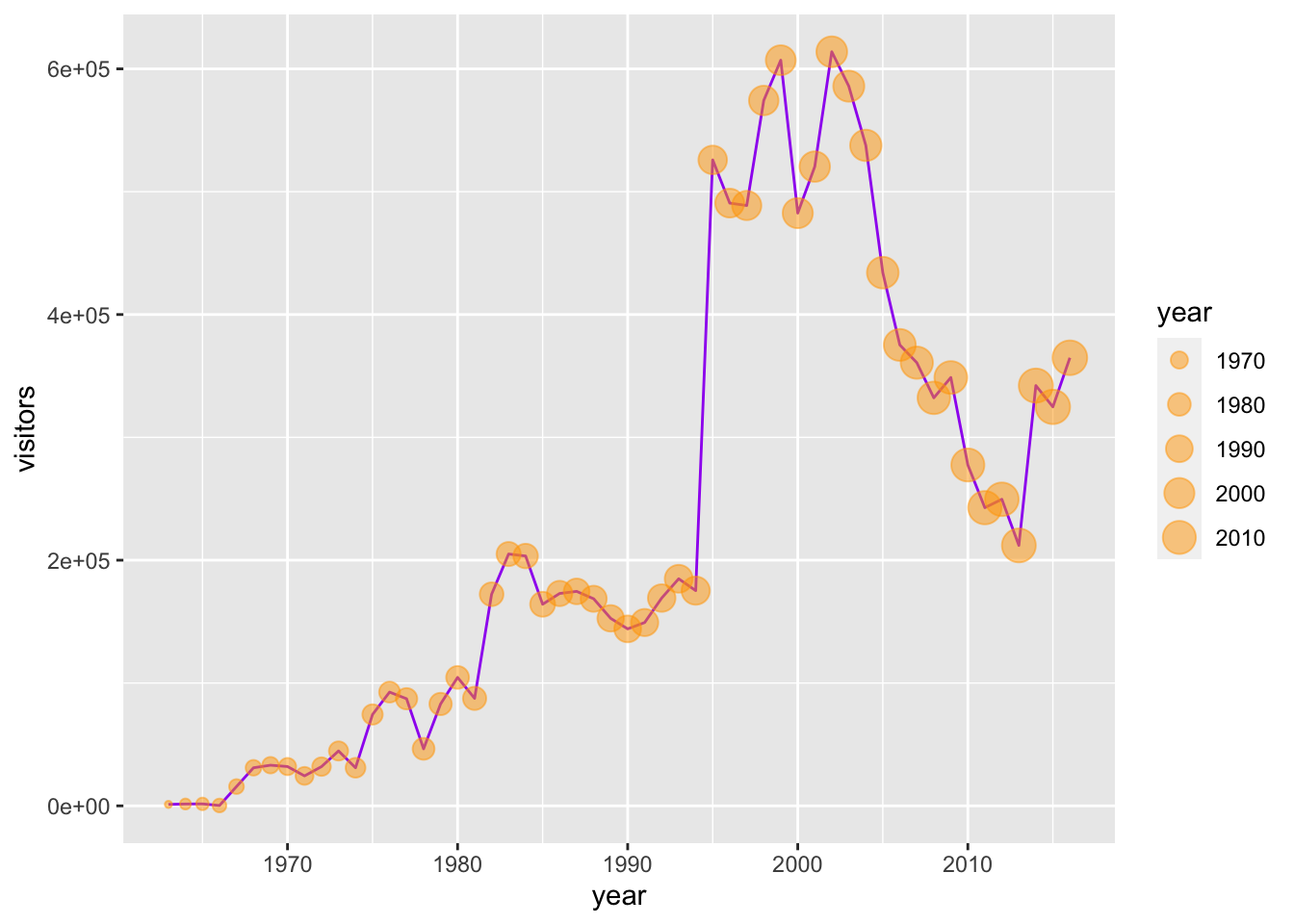Or, combine a column and line graph (not sure why you’d want to do this, but you can):

``````gg_base +
geom_col(fill = "orange",
color = "purple") +
geom_line(color = "green")``````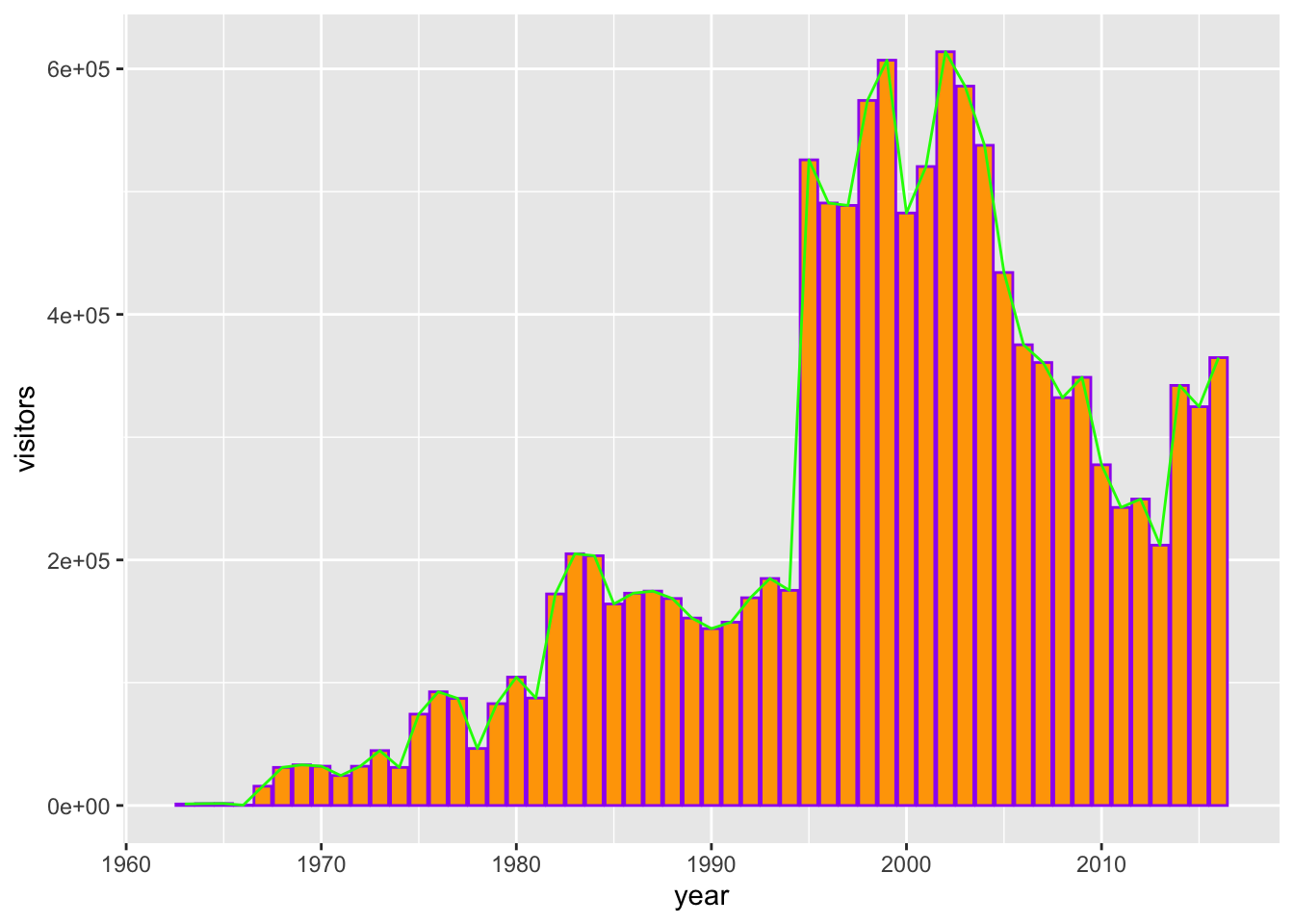## 5.10 Multi-series ggplot graphs

In the examples above, we only had a single series - visitation at Channel Islands National Park. Often we’ll want to visualize multiple series. For example, from the `ca_np` object we have stored, we might want to plot visitation for all California National Parks.

To do that, we need to add an aesthetic that lets `ggplot` know how things are going to be grouped. A demonstration of why that’s important - what happens if we don’t let ggplot know how to group things?

``````ggplot(data = ca_np, aes(x = year, y = visitors)) +
geom_line()``````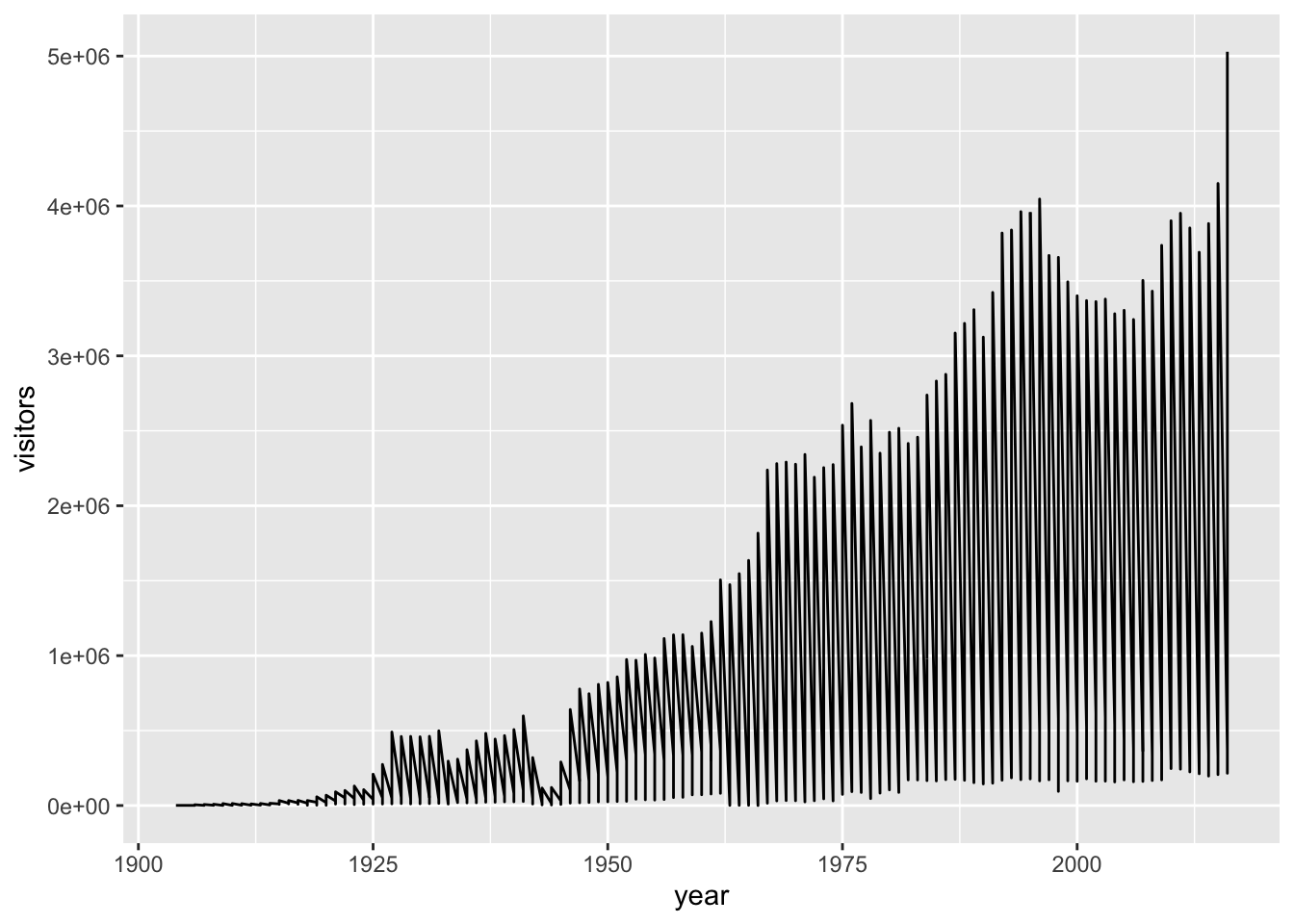Well that’s definitely a mess, and it’s because ggplot has no idea that these should be different series based on the different parks that appear in the ‘park_name’ column.

We can make sure R does know by adding an explicit grouping argument (`group =`), or by updating an aesthetic based on park_name:

``````ggplot(data = ca_np, aes(x = year, y = visitors, group = park_name)) +
geom_line()``````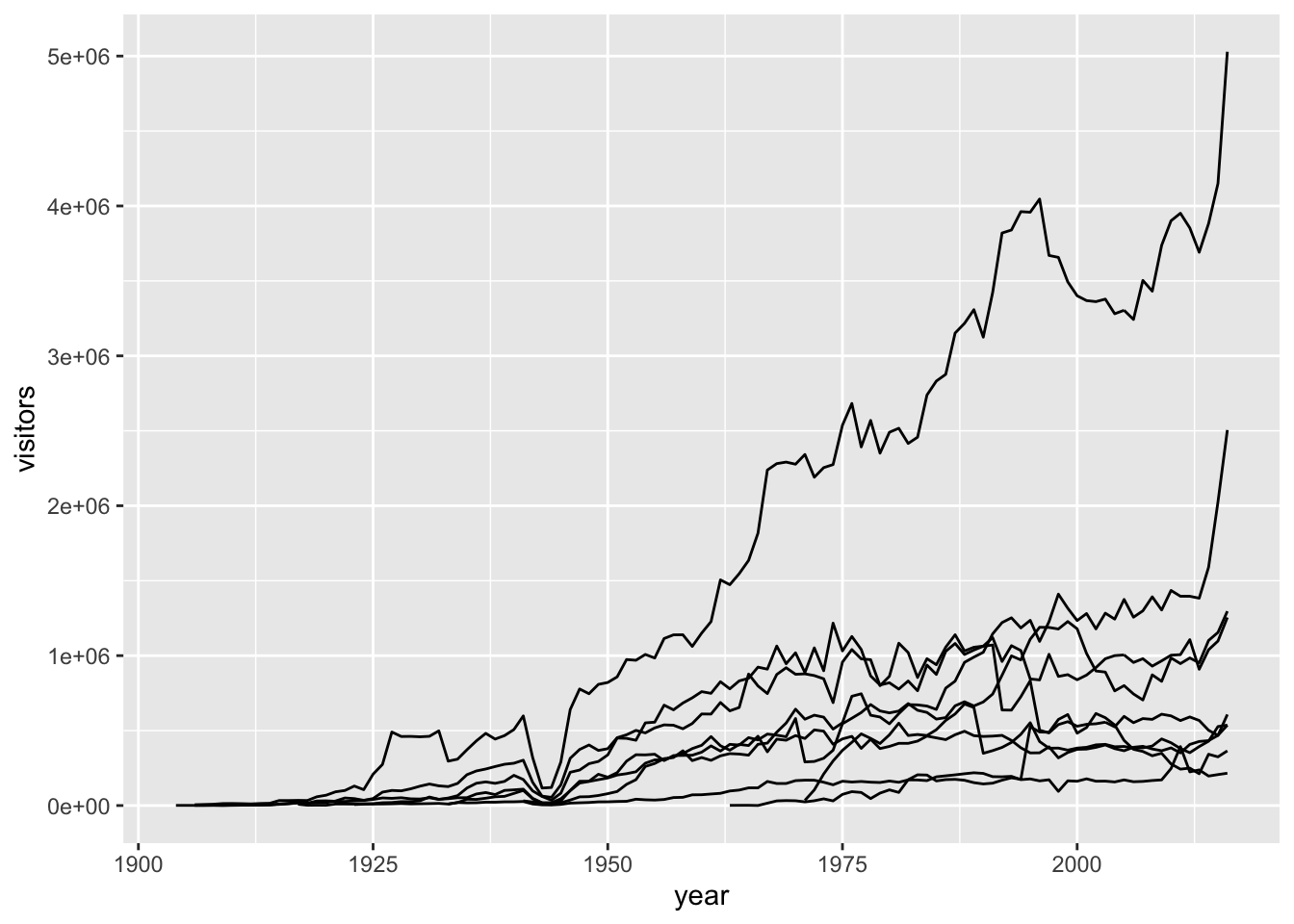Note: You could also add an aesthetic (`color = park_name`) in the `geom_line()` layer to create groupings, instead of in the topmost `ggplot()` layer.

Let’s store that topmost line so that we can use it more quickly later on in the lesson:

``gg_np <- ggplot(data = ca_np, aes(x = year, y = visitors, group = park_name))``

## 5.11 Faceting ggplot graphs

When we facet graphs, we split them up into multiple plotting panels, where each panel contains a subset of the data. In our case, we’ll split the graph above into different panels, each containing visitation data for a single park.

Also notice that any general theme changes made will be applied to all of the graphs.

``````gg_np +
geom_line(show.legend = FALSE) +
theme_light() +
labs(x = "year", y = "annual visitors") +
facet_wrap(~ park_name)``````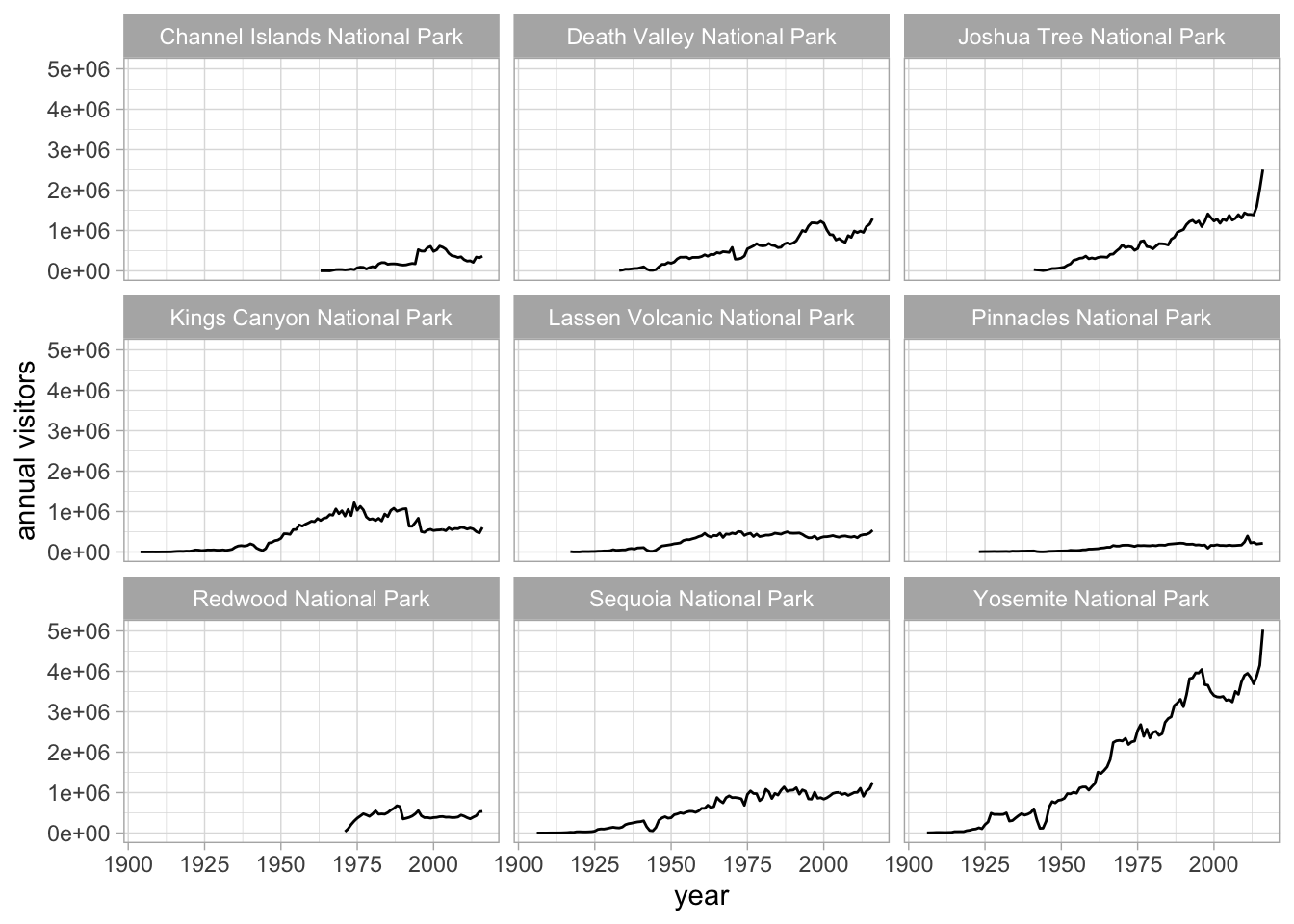## 5.12 Exporting a ggplot graph with `ggsave()`

If we want our graph to appear in a knitted html, then we don’t need to do anything else. But often we’ll need a saved image file, of specific size and resolution, to share or for publication.

`ggsave()` will export the most recently run ggplot graph by default (`plot = last_plot()`), unless you give it the name of a different saved ggplot object. Some common arguments for `ggsave()`:

• `width =`: set exported image width (default inches)
• `height =`: set exported image height (default height)
• `dpi =`: set dpi (dots per inch)

So to export the faceted graph above at 180 dpi, width a width of 8" and a height of 7", we can use:

``ggsave(here("figures", "np_graph.jpg"), dpi = 180, width = 8, height = 7)``

Notice that a .jpg image of that name and size is now stored in the `figures\` folder within your working directory. You can change the type of exported image, too (e.g. pdf, tiff, eps, png, mmp, svg).

Sync your project with your GitHub repo.

• Stage
• Commit
• Pull (to check for remote changes)
• Push!